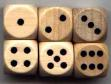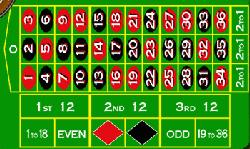# Probability and odds in gambling

• Probability
• Odds
• Odds and probabilities resources online

## Probability

Understanding the nature of simple probability is key to understanding all matters gambling-related. In gambling terms, we define the probability of a winning event as follows:

 The number of ways of winning divided by the total number of possible outcomes

As a practical example, let's look at that doyen of gambling tools, the six-sided die:There are six possible outcomes - each one of the six sides. The probability of any one side is therefore one divided by six. Probability is always expressed as a number between zero and one, so for this example, 1 ÷ 6 = 0.166.

We can also make "0.166" a bit more meaningful by multiplying by 100 and expressing it as a percentage: 0.166 × 100 = 16.6%.

Equally, though, you can simply express it as "one in six"

As a further example, another piece of gambling equipment that's stood the test of time: the roulette table:There are 37 numbers in total. The probability of the little ivory ball falling into any one of the 37 compartments is therefore 1 ÷ 37 = 0.027, or 2.7%.

The same applies to any of the other vast array of bets offered in roulette. For example: the "dozens" bets ("1st 12"...etc) have 12 ways of winning out of the 37 total; 12 ÷ 37 = 0.32, or 32%.

## Odds

"Odds" is only a very marginally different concept to probability; probability requires that we look at the event in question in relation to the total number of events; when calculating odds, however, we take the event against all the other events, exclusive of the event itself.

Going back to the dice example above: the odds for any one of the six sides are the side itself against the five other sides. Odds being expressed in ratio format rather than as a decimal, this gives us odds of 1:5 ("one to five") - as opposed to the probability, 1/6.

Odds can be expressed both "for" and "against"; the odds "against" the event in question is simply a reversal of the odds "for"; as such, the odds against any of the six sides is expressed as 5:1.

Looking back at the roulette table: the odds for any one number are the number itself against the 36 other numbers, 1:36, or 36:1 against.

Things get a little more complicated when we start dealing with more than one event, such as the "dozen" bets, because we need to subtract those extra winning numbers from the overall total; 37 - 12 = 25, so the odds for the dozen bets are 12:25. The "even money" bets, red/black, high/low etc, come in at 18:19 - eighteen winning bets and nineteen losers, the extra loser coming as a result of the "zero" compartment.

The concept of odds is crucial to your understanding of how casino games work: once you get your head around the idea of "the winning event against the losing alternatives", you can start to apply it in relation to the payoffs that the casino offers on those bets - and this leads us to the "house edge", the single most important consideration regarding your financial well being in the casino.

### Probability and odds resources online

Some useful external resources and articles about probability and odds.

Wizard Of Odds: Dice probability
Wizard Of Odds: Dice probability questions
Wizard Of Odds: Card probability questions
Wizard Of Odds: Coin probability questions
Wizard Of Odds: Probabilities for the sum of 1 to 25 dice